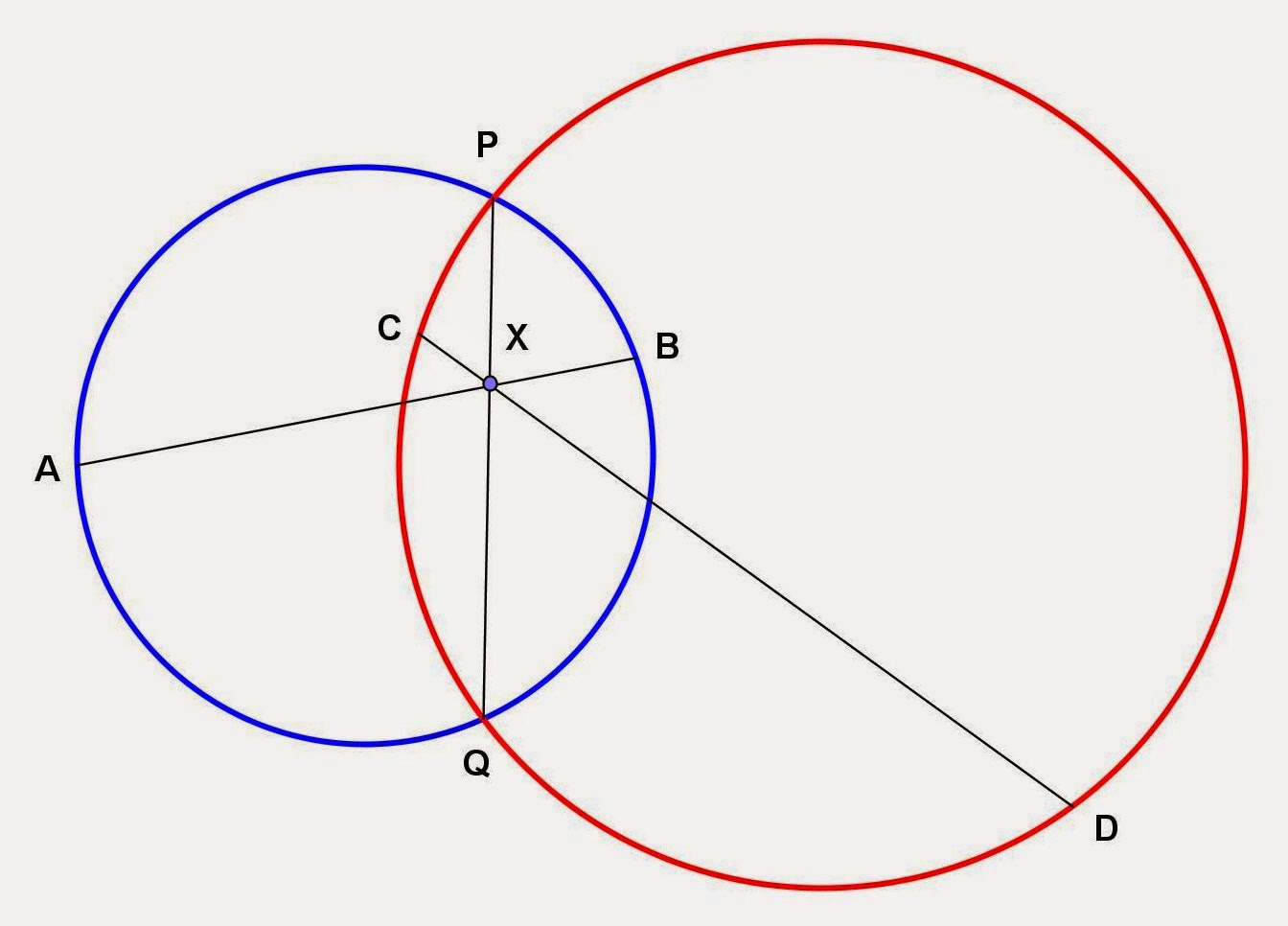Friday, December 19, 2014

Circle and Secant Segment Problems, 5

Category: Plane Geometry

"Published in Newark, California, USA"

Draw two intersecting circles with common chord PQ and let X be any point on PQ. Through X draw any chord AB of one circle. Also draw through X any chord CD of the other circle. Prove that AX • XB = CX • XD.

Solution:

In the given word problem, you can draw two intersecting circles of any size you wish and then label further the figure while analyzing the word problem as followsPhoto by Math Principles in Everyday Life

If a theorem says "When two chords intersect inside a circle, the product of the segments of one chord equals the product of the segments of the other chord.", then the working equation for the small circle is

and for the big circle is

Since PQ is the common chord of two circles, then we can equate the two working equations as follows

Therefore,